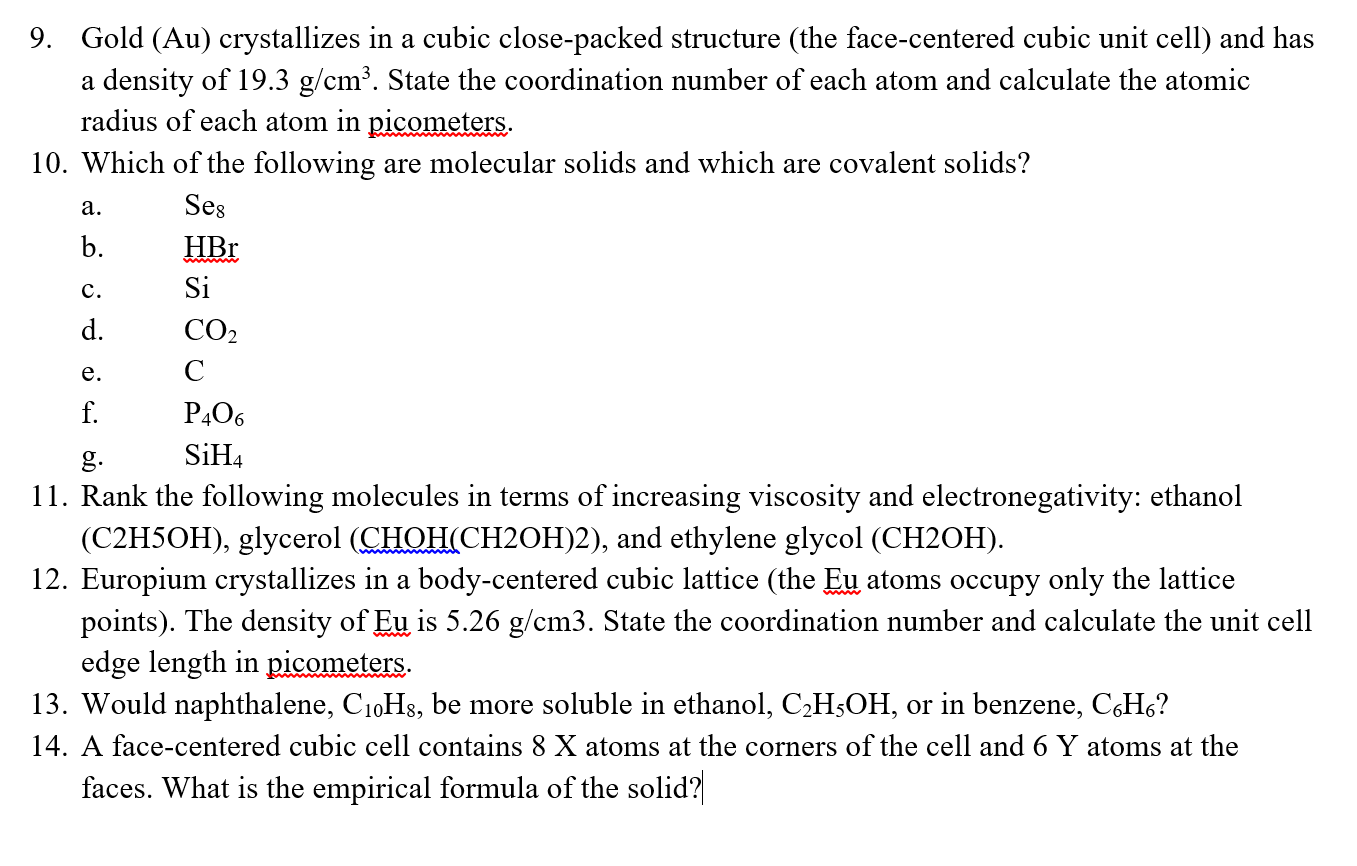# Gold (Au) crystallizes in a cubic close-packed structure (the face-centered cubic unit cell) and hasa density of 19.3 g/cm3. State the coordination number of each atom and calculate the atomicradius of each atom in picometers.9.10. Which of the following are molecular solids and which are covalent solids?Sesa.b. HBrC.d. CO2e. Cf.SiP406g. SiH(C2H5OH), glycerol (CHOH CH20H)2), and ethylene glycol (CH20H).points). The density of Eu is 5.26 g/cm3. State the coordination number and calculate the unit cell11. Rank the following molecules in terms of increasing viscosity and electronegativity: ethanol12. Europium crystallizes in a body-centered cubic lattice (the Eu atoms occupy only the latticeedge length in picometers.13. Would naphthalene, CioHs, be more soluble in ethanol, C2HsOH, or in benzene, CsHs?14. A face-centered cubic cell contains 8 X atoms at the corners of the cell and 6 Y atoms at thefaces. What is the empirical formula of the solid

Question
482 views

Need help with numbers 9 to 14. Thanks!help_outlineImage TranscriptioncloseGold (Au) crystallizes in a cubic close-packed structure (the face-centered cubic unit cell) and has a density of 19.3 g/cm3. State the coordination number of each atom and calculate the atomic radius of each atom in picometers. 9. 10. Which of the following are molecular solids and which are covalent solids? Ses a. b. HBr C. d. CO2 e. C f. Si P406 g. SiH (C2H5OH), glycerol (CHOH CH20H)2), and ethylene glycol (CH20H). points). The density of Eu is 5.26 g/cm3. State the coordination number and calculate the unit cell 11. Rank the following molecules in terms of increasing viscosity and electronegativity: ethanol 12. Europium crystallizes in a body-centered cubic lattice (the Eu atoms occupy only the lattice edge length in picometers. 13. Would naphthalene, CioHs, be more soluble in ethanol, C2HsOH, or in benzene, CsHs? 14. A face-centered cubic cell contains 8 X atoms at the corners of the cell and 6 Y atoms at the faces. What is the empirical formula of the solid fullscreen
check_circle

Step 1

Dear student, you have posted multiple questions in a single file. I am answering the first one. Kindly re-post other questions each in single file.

The coordination number of each atom in face-centred cubic arrangement is 12.

Step 2
Step 3

### Want to see the full answer?

See Solution

#### Want to see this answer and more?

Solutions are written by subject experts who are available 24/7. Questions are typically answered within 1 hour.*

See Solution
*Response times may vary by subject and question.
Tagged in